# Math in Focus Grade 2 Cumulative Review Chapters 1 to 4 Answer Key

Go through the Math in Focus Grade 2 Workbook Answer Key Cumulative Review Chapters 1 to 4 to finish your assignments.

## Math in Focus Grade 2 Cumulative Review Chapters 1 to 4 Answer Key

Concepts and Skills

Write in standard form.

Question 1.
Seven hundred sixteen _______
716.

Explanation:
The standard form of Seven hundred sixteen is 716.

Question 2.
Four hundred five _______
405.

Explanation:
The standard form of Four hundred five is 405.

Count on or count back. Find the missing numbers.

Question 3.
820, 810, 800, ___, ___, ____
820, 810, 800, 790, 780, 760.

Explanation:
The missing numbers are 820, 810, 800, 790, 780, 760. As we can see the countback is reduced to 10.

Question 4.
600, 700, 800, ___, _____
600, 700, 800, 900, 1000.

Explanation:
The missing numbers are 600, 700, 800, 900, 1000. As we can see the count on adds 100.

Question 5.
500, 400, 300, ___, ___, ____
500, 400, 300, 200, 100.

Explanation:
The missing numbers are 500, 400, 300, 200, 100. As we can see the countback is reduced to 100.

Find the missing numbers.

Question 6.
In 632, the digit ____ is in the tens place.
3.

Explanation:
Here, in the number 632, the digit 3 is in the tens place.

Question 7.
In 591, the digit 5 is in the ____ place.
Hundreds place.

Explanation:
Here, in the number 591, the digit 5 is in the hundreds place.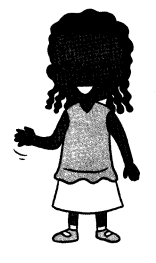Question 8.
743 = 700 + ___ + 3
40.

Explanation:
The expanded form of 743 is 700+40+3, so the missing digit is 40.

Question 9.
200 and 2 make ___
202.

Explanation:
Here, 200 and 2 make 202.

Write > or <.

Question 10.
235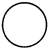325
235 < 325.

Explanation:
In the above, we can see that 235 is less than 325.
235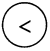325

Question 11.
891889
891 > 889.

Explanation:
In the above, we can see that 891 is greater than 889.
891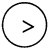889

Order the numbers from least to greatest.

Question 12.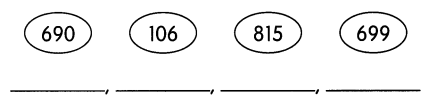106, 690, 699, 815.

Explanation:
In the above, the numbers from least to greatest are 106, 690, 699, 815.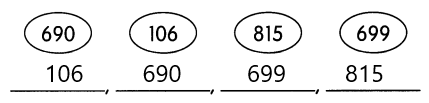Find the missing numbers.

Question 13.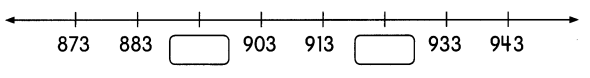893, 923.

Explanation:
As we can see the numbers are adding 10 to the next numbers in the number line. The number line will be 873, 883, 893, 903, 913, 923, 933, 943.  So the missing numbers are 893, 923.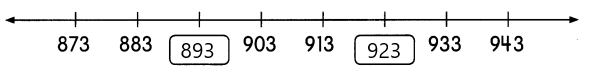Fill in the blanks.

Question 14.
1 more than 638 is ___
1 more than 638 is 639.

Explanation:
Here, 1 more than 638 is 638+1 which is 639.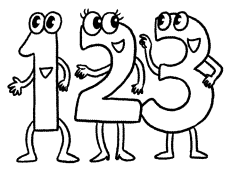Question 15.
10 less than 286 is ___
10 less than 286 is 276.

Explanation:
Here, 10 less than 286 is 286-10 which is 276.

Question 16.
100 more than 899 is ____
100 more than 899 is 999.

Explanation:
Here, 100 more than 899 is 899+100 which is 999.

Question 17.
____ is 1 less than 360.
359 is 1 less than 360.

Explanation:
Here, 1 less than 360 is 360-1 which is 359.

Question 18.
____ is 10 more than 890.
900 is 10 more than 890.

Explanation:
Here, 10 more than 890 is 890+10 which is 900.

Question 19.
___ is 100 less than 1,000.
900 is 100 less than 1,000.

Explanation:
Here, 100 less than 1,000 is 1,000-100 which is 900.

Complete each pattern.

Question 20.
240, 220, ___, ___, 160, ____, ____.
240, 220, 200, 180, 160, 140, 120.

Explanation:
In the above, we can see that the pattern is 20 is less than countback. So the pattern is 240, 220, 200, 180, 160, 140, 120.

Question 21.
350, 390, 430, __, ___, ___, ____
350, 390, 430, 470, 510, 550, 590.

Explanation:
In the above, we can see that the pattern is 40 is greater than the count on. So the pattern is 350, 390, 430, 470, 510, 550, 590.

Question 22.
___, ___, 454, 354, 254, __, ____
654, 554, 454, 354, 254, 154, 54.

Explanation:
In the above, the pattern is 654, 554, 454, 354, 254, 154, 54. Here the numbers are less than 100.

Question 23.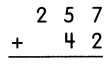257+42 = 299.

Explanation:
The addition of 257 and 42 is 299.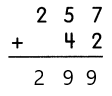Question 24.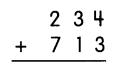234+713 = 947.

Explanation:
The addition of 234 and 713 is 947.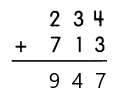Question 25.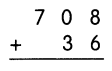708+36 = 744.

Explanation:
The addition of 708 and 36 is 744.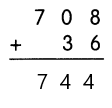Question 26.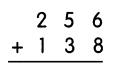256+138 = 394.

Explanation:
The addition of 256 and 138 is 394.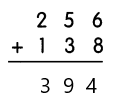Question 27.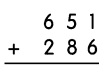651+286 = 937.

Explanation:
The addition of 651 and 286 is 937.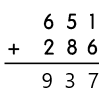Question 28.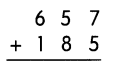657+185 = 842.

Explanation:
The addition of 657 and 185 is 842.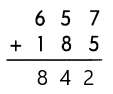Subtract.

Question 29.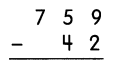759-42 = 717
717+42 = 759.

Explanation:
The subtraction of 759 and 42 is 717. So to check that the answer is correct we will add the result with subtrahend. So 717+42 is 759.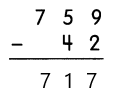Question 30.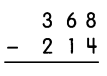368-214 = 154,
154+214 = 368.

Explanation:
The subtraction of 368 and 214 is 154. So to check that the answer is correct we will add the result with subtrahend. So 154+214 is 368.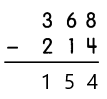Question 31.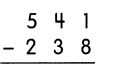541-238 = 303,
303+238 = 541.

Explanation:
The subtraction of 541 and 238 is 303. So to check that the answer is correct we will add the result with subtrahend. So 303+238 is 541.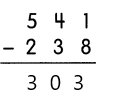Question 32.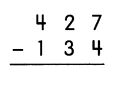427-134 = 293,
293+134 = 427.

Explanation:
The subtraction of 427 and 134 is 293. So to check that the answer is correct we will add the result with subtrahend. So 293+134 is 427.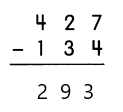Question 33.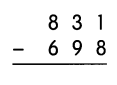831-698 = 133,
133+698 = 831.

Explanation:
The subtraction of 831 and 698 is 133. So to check that the answer is correct we will add the result with subtrahend. So 133+698 is 831.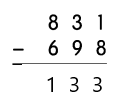Question 34.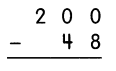200-48 = 152,
152+48 = 200.

Explanation:
The subtraction of 200 and 48 is 152. So to check that the answer is correct we will add the result with subtrahend. So 152+48 is 200.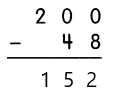Subtract.

Question 37.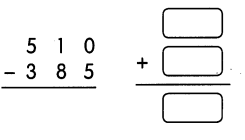510-385 = 125,
125+385 = 510.

Explanation:
The subtraction of 510 and 385 is 125. So to check that the answer is correct we will add the result with subtrahend. So 125+385 is 510.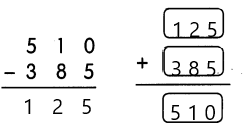Question 38.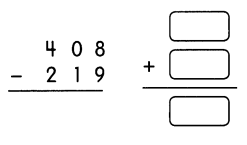408-219 = 189,
189+219 = 408.

Explanation:
The subtraction of 408 and 219 is 189. So to check that the answer is correct we will add the result with subtrahend. So 189+219 is 408.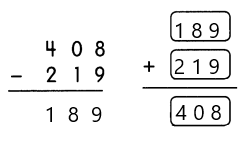Find the missing numbers.

Question 39.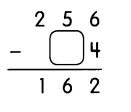The missing number is 9.

Explanation:
Here, we have a minuend of 256 and the difference is 162. So to find the missing number we will subtract the minuend with the product and 256-162 which is 94, so the missing number is 9.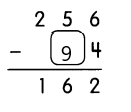Question 40.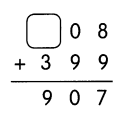The missing number is 5.

Explanation:
Here, we have an addend as 399 and the sum is 907. So to find the missing number we will subtract the sum with the addend and 907-399 which is 508, so the missing number is 5.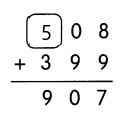Problem Solving

Solve.

Question 41.
Manuel drives 215 miles on Monday. He drives 685 miles on Tuesday. How many miles does he drive in all?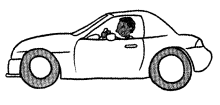He drives _________ miles in all.
He drives 900 miles in all.

Explanation:
Given that Manuel drives 215 miles on Monday and he drives 685 miles on Tuesday. So the number of miles does he drive in all is 215+685 which is 900 miles.

Question 42.
Mrs. King has $200 in the bank. She spends$45. How much does she have left?
She has $___ left. Answer: She has$155 left.

Explanation:
Given that Mrs. King has $200 in the bank and she spends$45. So the money left with Mrs. King is $200 –$45 = \$155.

Solve.

Question 43.

Explanation:
Given that Jeremy has 30 black beads and he has 50 more red beads than black beads. So the red beads does he have is 30+50 which is 80 red beads.

Question 44.
There are 356 sheep on a farm. There are 100 fewer cows than sheep. How many cows are there?
There are ____ cows.
There are 256 cows.

Explanation:
Given that there are 356 sheep on a farm and there are 100 fewer cows than sheep. So the number of cows are there on the farm is 356-100 which is 256 cows.

Question 45.
Mike has 515 stickers in his album. Shateel has 488 stickers in his. Who has more stickers? How many more stickers?
___ has more stickers.
___ more stickers.
Mike has more stickers.
27 more stickers.

Explanation:
Given that Mike has 515 stickers in his album and Shateel has 488 stickers in his. So the number of more stickers are 515-488 which is 27 stickers Mike has more.

Solve.

Question 46.
Nick scores 715 points in a game. He scores 100 fewer points than his sister. How many points does his sister score?
His sister scores ___ points.
His sister scores 615 points.

Explanation:
Given that Nick scores 715 points in a game and he scores 100 fewer points than his sister. So the number of points does his sister score is 715-100 which is 615 points.

Question 47.
Beth reads for 60 minutes in the morning. She reads for 42 minutes at night. How many minutes does she read in all?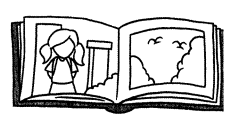Beth reads for ___ minutes in all.
Beth reads for 42 minutes in all.

Explanation:
Given that Beth reads for 60 minutes in the morning and she reads for 42 minutes at night. So the number of minutes does she read in all is 60+42 which is 102 minutes.

Question 48.
339 passengers are on a train. 196 of them are children. The others are adults. How many adults are on the train?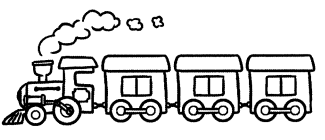There are ___ adults on the train.
There are 143 adults on the train.

Explanation:
Given that there are 339 passengers are on a train and 196 of them are children and the others are adults. So the number of adults are on the train is 339-196 which is 143 adults.

Question 49.
The Hat Store sold 265 caps last week. It sold 97 fewer caps this week.

a. How many caps did the Hat Store sell this week?

b. How many caps did the Hat Store sell for the two weeks?

a. The Hat Store sold ___ caps this week.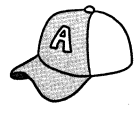b. The Hat Store sold ___ caps for the two weeks.

The Hat Store sold 168 caps this week.
The Hat Store sold 336 caps for the two weeks.

Explanation:
Given that the hat store sold 265 caps last week and it sold 97 fewer caps this week. So the number of caps did the hat store sell this week is 265-97 which is 168 hats. So the hat store sold 168 caps this week. For two weeks the hat store sold 168×2 which is 336 caps.

Solve.

Question 50.
The theater sold 343 tickets on Friday. This is 192 fewer tickets than those sold on Saturday. How many tickets were sold altogether?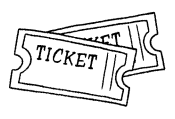____ tickets were sold altogether.